## UGC NET CS 2018 JUNE Paper-2

 Question 1
The definitions in an XML document are said to be when the tagging system and definitions in the DTD are all in compliance.
 A well-formed B reasonable C valid D logical
Web-Technologies       XML
Question 1 Explanation:
→ A XML document is said to be well- formed if it have correct syntax like tags are case sensitive, elements must have closing tags, elements must be properly nested etc.
→ But when when associate a well formed XML document with a DTD( in which we define the structure of a document) and if that XML document validates all the definitions defined in DTD then that XML document is called as “valid” XML document.
→ Always remember that a “valid” XML document is also a “well-formed” XML document but a “well-formed” XML document is not necessarily a “valid” XML document
→ In question, it is mentioned that XML document is validating the definitions in the DTD. so the answer is option (3).
 Question 2
Consider the JavaScript Code :
var y= ’’12”;
function f( )
{
var y=’’6”;
function g( )
{
}
g( );
}
f( );
If M is the number of alert dialog boxes generated by this JavaScript code and D1, D2, ...., DM represents the content displayed in each of the M dialog boxes, then :
 A M=3; D1 displays ”12”; D2 displays ”6”; D3 displays ”12”. B M=3; D1 displays ”6”; D2 displays ”12”; D3 displays ”6”. C M=2; D1 displays ”6”; D2 displays ”12”. D M=2; D1 displays ”12”; D2 displays ”6”.
Web-Technologies       Java-Script
Question 2 Explanation:
The JavaScript this keyword refers to the object it belongs to.
It has different values depending on where it is used:
In a method, this refers to the owner object.
Alone, this refers to the global object.
In a function, this refers to the global object.
In an event, this refers to the element that received the event.
Methods like call(), and apply() can refer this to any object.
There are two alert boxes in the javascript code.So two messages will be displayed.
First message, alert(this.y) here this.y is global variable whose value is 12 So first message is “12”.
Second message is alert(y) , here “y” local variable and value is 6 so second message displays value “6”
 Question 3
What is the output of the following JAVA program ?
class simple
{
public static void main(String[ ] args)
{
simple obj = new simple( );
obj.start( );
}
void start( )
{
long [ ] P= {3, 4, 5};
long [ ] Q= method (P);
System.out.print (P + P + P+”:”);
System.out.print (Q + Q + Q);
}
long [ ] method (long [ ] R)
{
R =7; return R;
}
} //end of class
 A 12 : 15 B 15 : 12 C 12 : 12 D 15 : 15
OOPS       JAVA
Question 3 Explanation:
First we will create the object of simple class.
By using object , we call the function start().
In the start() function definition, first statement is integer array with three elements.
long [ ] Q= method (P); Again function method(p) will be called.
In the definition of method function, we are changing the second element of array to value 7 and returning updated array to array Q.
We are passing address of P as argument to method so Modifications happened in the method automatically reflects to array P.
Both array P and Q consists of values {3,7,5}
The sum of the three values are 15
 Question 4
What is the output of the following ‘C’ program ? (Assuming little - endian representation of multi-byte data in which Least Significant Byte (LSB) is stored at the lowest memory address.)
#include
#include
/* Assume short int occupies two bytes of storage */
int main ( )
{
union saving
{
short int one;
char two;
};
union saving m;
m.two  = 5;
m.two  = 2;
printf(’’%d, %d, %d\n”, m.two , m.two , m.one);
}/* end of main */
 A 5, 2, 1282 B 5, 2, 52 C 5, 2, 25 D 5, 2, 517
Programming       Structure
Question 4 Explanation:
● m.two holds the value 5 and m.two holds the value 2 So it will print 5 and 2 values.
● Size of the short integer is 2 bytes. Saving is union variable, we will access one variable at time and only one memory location is shared among all the members.So the two values 5 and 2 (two bytes of the data) will store in little endian format in the variable m.one.
● Endianness is the sequential order in which bytes are arranged into larger numerical values when stored in memory or when transmitted over digital links.
● In big-endian format, whenever addressing memory or sending/storing words bytewise, the most significant byte—the byte containing the most significant bit—is stored first (has the lowest address) or sent first, then the following bytes are stored or sent in decreasing significance order,
● Little-endian format reverses this order: the sequence addresses/sends/stores the least significant byte first (lowest address) and the most significant byte last (highest address).
● First 5 will store in the lowest address and 2 will store next highest address.
● So the binary representation 5 and 2 in little endian format is 00000010 00000101
The binary number of the above is 517
 Question 5
Given below are three implementations of the swap( ) function in C++ :Which of these would actually swap the contents of the two integer variables p and q ?
 A (a) only B (b) only C (c) only D (b) and (c) only
Programming       Functions
Question 5 Explanation:
Module -(a) is call by value , so by using that code w can not swap the contents.
Module -(b) is call by reference , So the modification of content in the function reflects the changes in the main program. Swapping is done in the module -(b).
Module -(c) is passing addresses as parameters but in the functions definition , We are changing the addresses not the content So swapping of the values can’t be done.
 Question 6
​In Java, which of the following statements is/are True ?
S1 : The ‘final’ keyword applied to a class definition prevents the class from being extended through derivation.
S2 : A class can only inherit one class but can implement multiple interfaces.
S3 : Java permits a class to replace the implementation of a method that it has inherited. It is called method overloading.
 A S1 and S2 only B S1 and S3 only C S2 and S3 only D All of S1, S2 and S3
OOPS       JAVA
Question 6 Explanation:
● If a class has multiple methods having same name but different in parameters, it is known as Method Overloading.Method Overloading is a feature that allows a class to have more than one method having the same name, if their argument lists are different.So the option-3 is False.
● The final keyword in java is used to restrict the user. The java final keyword can be used in many context. Final can be: variable, method and class.
● A Java class can only extend one parent class. Multiple inheritance (extends) is not allowed. Interfaces are not classes, however, and a class can implement more than one interface.
 Question 7
Which of the following statements is/are True ?
P : C programming language has a weak type system with static types.
Q : Java programming language has a strong type system with static types.
 A P only B Q only C Both P and Q D Neither P nor Q
Programming       Storage-Classes
Question 7 Explanation:
→ A strongly typed language has stricter typing rules at compile time, which implies that errors and exceptions are more likely to happen during compilation. Most of these rules affect variable assignment, return values and function calling.
→ A weakly typed language has looser typing rules and may produce unpredictable results or may perform implicit type conversion at runtime
→ Java, C#, Ada and Pascal are sometimes said to be more strongly typed than C, a claim that is probably based on the fact that C supports more kinds of implicit conversions, and C also allows pointer values to be explicitly cast while Java and Pascal do not.
→ Java itself may be considered more strongly typed than Pascal as manners of evading the static type system in Java are controlled by the Java virtual machine's type system.
→ C# and VB.NET are similar to Java in that respect, though they allow disabling of dynamic type checking by explicitly putting code segments in an "unsafe context".
→ Pascal's type system has been described as "too strong", because the size of an array or string is part of its type, making some programming tasks very difficult
 Question 8
A graphic display system has a frame buffer that is 640 pixels wide, 480 pixels high and 1 bit of color depth. If the access time for each pixel on the average is 200 nanoseconds, then the refresh rate of this frame buffer is approximately :
 A 16 frames per second B 19 frames per second C 21 frames per second D 23 frames per second
Computer-Organization       Graphics
Question 8 Explanation:
Given data,
- Width (or) wide =640 pixels
- Height (or) High =480 pixels
- Color depth =1 bit/pixel
- Access time of each pixel on the average =200ns
- Refresh rate of frame buffer=?
Step-1: Graphic display system =640*480
=307200
Step-2: Memory required for Graphic display system =640*480*1 =307200
Step-3: Total screen access time = Memory required for Graphic display system * Access time of each pixel
= 307200*200 ns
= 61440000 ns
Step-4: Refresh rate of frame buffer per second=(10​ -9​ )/61440000
= 16.27604166 frames per second
[ Note: 10​ -9​ =1000000000 ]
 Question 9
Which of the following statements is/are True regarding the solution to the visibility problem in 3D graphics ?
S1 : The Painter’s algorithm sorts polygons by depth and then paints (scan - converts) each Polygon onto the screen starting with the most nearest polygon.
S2 : Backface Culling refers to eliminating geometry with back facing normals.
 A S1 only B S2 only C Both S1 and S2 D Neither S1 Nor S2
Computer-Organization       Graphics
Question 9 Explanation:
Visibility problem in 3D graphics
1. Painter's algorithm
-A depth sorting method
-Surfaces are sorted in the order of decreasing depth
-Surfaces are drawn in the sorted order, and overwrite the pixels in the frame buffer
-Subtle difference from depth buffer approach: entire face drawn
-Two problems:
1. It can be nontrivial to sort the surfaces
2. There can be no solution for the sorting order

2. Back Face Culling
-Back faces: faces of opaque object which are “pointing away” from viewer
-Back face culling – remove back faces (supported by OpenGL)
How to detect back faces
-If we find backface, do not draw, save rendering resources
-There must be other forward face(s) closer to eye
-F is face of object we want to test if backface
-P is a point on F
-Form view vector, V as (eye – P)
-N is normal to face F
3. View-Frustum Culling
-Remove objects that are outside the viewing frustum
-Done by 3D clipping algorithm (e.g. Liang-Barsky)
4. Ray Tracing
-Ray tracing is another example of image space method
-Ray tracing: Cast a ray from eye through each pixel to the world.
5. Z(Depth buffer algorithm)
 Question 10
Consider the matrixrepresenting a set of planar (2D) geometric transformations in homogeneous coordinates.
Which of the following statements about the matrix M is True ?
 A M represents first, a scaling of vector (2, 1) followed by translation of vector (1, 1) B M represents first, a translation of vector (1, 1) followed by scaling of vector (2, 1) C M represents first, a scaling of vector (3, 1) followed by shearing of parameters (−1, 1) D M represents first, a shearing of parameters (−1, 1) followed by scaling of vector (3, 1)
Engineering-Mathematics       Vectors
Question 10 Explanation:
Scale Matrix:
The scale matrix has all the same zeros as the identity matrix, but it doesn’t necessarily keep using the ones across the diagonal. You are trying to decide how to scale your coordinate, and you don’t want the default scale value to be 1. Here is the scale matrix:
[
Sx 0 0 0
0 Sy 0 0
0 0 Sz 0
0 0 0 1
]
Translation Matrix
The translation matrix looks the same as the identity matrix, but the last column is a little different. The last column applies an amount of change for the x, y, and z coordinates:
[
1 0 0 Tx
0 1 0 Ty
0 0 1 Tz
0 0 0 1
]
 Question 11
Assume the following regarding the development of a software system P:
- Estimated lines of code of P : 33, 480 LOC
- Average productivity for P : 620 LOC per person-month
- Number of software developers : 6
- Average salary of a software developer : 50,000 per month
If E, D and C are the estimated development effort (in person-months), estimated development time (in months), and estimated development cost (in Lac) respectively, then (E, D, C) =
 A (48, 8, 24) B (54, 9, 27) C (60, 10, 30) D (42, 7, 21)
Software-Engineering       Software-design
Question 11 Explanation:
- Estimated lines of code of P : 33480 LOC
- Average productivity for P : 620 LOC per person-month
- Number of software developers : 6
- Average salary of a software developer : 50000 per month
Step-1: Estimated development effort (in person-months)= 33480 /620
E=54
Step-2: Estimated development time (in months) =54/6
D= 9 months
Step-3: Estimated development cost (in Lac) = 50000*6*9
=27 lacs
 Question 12
Match the following in Software Engineering :A (a)-(ii), (b)-(iii), (c)-(iv), (d)-(i) B (a)-(iii), (b)- (i), (c)-(iv), (d)-(ii) C (a)-(iv), (b)- (i), (c)-(ii), (d)-(iii) D (a)-(iii), (b)- (iv), (c)-(i), (d)-(ii)
Software-Engineering       Software-requirements
Question 12 Explanation:
→ Coupling and Cohesion are used in software design. Cohesion measures strength of a module while coupling measures interdependency between modules.
→ Software cost estimation must be done more diligently throughout the project life cycle so that in the future there are fewer surprises and unforeseen delays in the release of a product.
→ Validation Technique→ Symbolic Execution
→ Software Requirements Definition→ Structured System Analysis
 Question 13
Which one of the following is not typically provided by Source Code Management Software ?
 A Synchronisation B Versioning and Revision history C Syntax highlighting D Project forking
Software-Engineering       Software-configuration-management
Question 13 Explanation:
Source Code Management Software is the management of changes to documents, computer programs, large web sites, and other collections of information. Changes are usually identified by a number or letter code, termed the "revision number", "revision level", or simply "revision".
→ Source Code Management Software includes
1. Synchronisation
2. Versioning and Revision history
3. Project forking
 Question 14
A software system crashed 20 times in the year 2017 and for each crash, it took 2 minutes to restart. Approximately, what was the software availability in that year ?
 A 96.9924% B 97.9924% C 98.9924% D 99.9924%
Software-Engineering       Software-Availability
Question 14 Explanation:
Software availability:​ It is a software used to ensure that systems are running and available most of the time. High availability is a high percentage of time that the system is functioning. It can be formally defined as (1 – (down time/ total time))*100%. Although the minimum required availability varies by task, systems typically attempt to achieve 99.999% (5-nines) availability. This characteristic is weaker than fault tolerance, which typically seeks to provide 100% availability, albeit with significant price and performance penalties.
Given data,
-Software crashed 20 times in the year 2017
-each crash=2 minutes to restart.
-Software availability=?
1 year=365 days = 365*1440 minutes =525600 minutes.
→ Crash time =20*2
=40 minutes
→ Software availability = (525600-40) / 525600
= 0.999924
= (0.999924 * 100)
= 99.9924 %
 Question 15
Match the 5 CMM Maturity levels/CMMI staged representations in List- I with their characterizations in List-II :A (a)-(iv), (b)-(v), (c)-(i), (d)-(iii), (e)-(ii) B (a)-(i), (b)-(ii), (c)-(iv), (d)-(v), (e)-(iii) C (a)-(v), (b)-(iv), (c)-(ii), (d)-(iii), (e)-(i) D (a)- (iv), (b)-(v), (c)-(ii), (d)-(iii), (e)-(i)
Question 15 Explanation:
Initial Stage: ​ There may not exist a plan or it may be abandoned.
Repeatable : ​ There’s a plan and people stick to it.
Defined : ​ The plan for a project comes from a template for plans.
Managed: ​ The plan uses processes that can be measured quantitatively.
Optimizing : Processes are improved quantitatively and continually.Question 16
Coupling is a measure of the strength of the interconnections between software modules. Which of the following are correct statements with respect to module coupling ?
P : Common coupling occurs when one module controls the flow of another module by passing it information on what to do.
Q : In data coupling, the complete data structure is passed from one module to another through parameters.
R : Stamp coupling occurs when modules share a composite data structure and use only parts of it.
 A P and Q only B P and R only C Q and R only D All of P, Q and R
Software-Engineering       Software-process-models
Question 16 Explanation:
Common Coupling:​ Common coupling occurs if two modules share same global data.
Data Coupling :​ Data coupling occurs when two modules communicate using elementary data items that are passed as parameters between two modules.
Stamp Coupling​ : Stamp coupling occurs if two modules communicate using composite items such as records in Pascal or structure in C
 Question 17
A software design pattern often used to restrict access to an object is :
 A adapter B decorator C delegation D proxy
Software-Engineering       Software-design
Question 17 Explanation:
Proxy pattern:​ a class functioning as an interface to another thing. In the proxy, extra functionality can be provided, for example caching when operations on the real object are resource intensive, or checking preconditions before operations on the real object are invoked.
→ For the client, usage of a proxy object is similar to using the real object, because both implement the same interface.
→ Proxy pattern solve the access to an object should be controlled and functionality should be provided when accessing an object.
→ When accessing sensitive objects, for example, it should be possible to check that clients have the needed access rights.
 Question 18
Reasons to re-engineer a software include :
P : Allow legacy software to quickly adapt to the changing requirements
R : Improve software maintainability
S : Allow change in the functionality and architecture of the software
 A P, R and S only B P and R only C P, Q and S only D P, Q and R only
Software-Engineering       Software-design
Question 18 Explanation:
→ Software re-engineering is the examination and alteration of a system to reconstitute it in a new form.​ It is done to improve the maintainability of the software.
→ Software reengineering encompasses inventory analysis, document restructuring, reverse engineering, program and data restructuring, and forward engineering. The intent of these activities is to create versions of existing programs that exhibit higher quality and better Maintainability.
A software reengineering process model:Question 19
Which of the following is not a key strategy followed by the clean room approach to software development ?
 A Formal specification B Dynamic verification C Incremental development D Statistical testing of the system
Software-Engineering       Software-Development
Question 19 Explanation:
The basic principles of the clean room process are
1. Software development based on formal methods: Software tool support based on some mathematical formalism includes model checking, process algebras, and Petri nets. The Box Structure Method might be one such means of specifying and designing a software product. Verification that the design correctly implements the specification is performed through team review, often with software tool support.
2. Incremental implementation under statistical quality control Cleanroom development uses an iterative approach, in which the product is developed in increments that gradually increase the implemented functionality. The quality of each increment is measured against pre-established standards to verify that the development process is proceeding acceptably. A failure to meet quality standards results in the cessation of testing for the current increment, and a return to the design phase.
3. Statistically sound testing
Software testing in the cleanroom process is carried out as a statistical experiment. Based on the formal specification, a representative subset of software input/output trajectories is selected and tested. This sample is then statistically analyzed to produce an estimate of the reliability of the software, and a level of confidence in that estimate.
 Question 20
Which of the following statements is/are True ?
P : Refactoring is the process of changing a software system in such a way that it does not alter the external behavior of the code yet improves the internal architecture.
Q : An example of refactoring is adding new features to satisfy a customer requirement discovered after a project is shipped.
 A P only B Q only C Both P and Q D Neither P nor Q
Software-Engineering       Software-design
Question 20 Explanation:
→ Refactoring allows a software engineer to improve the internal structure of a design (or source code) without changing its external functionality or behavior of the code yet improves the internal structure. In essence, refactoring can be used to improve the efficiency, readability, or performance of a design or the code that implements a design.
→ It is a disciplined way to clean up code [and modify/simplify the internal design] that minimizes the chances of introducing bugs. In essence, when you refactor you are improving the design of the code after it has been written.
Note: It won’t add any new featuring to satisfy a customer requirement. So, statement Q is false.
 Question 21
The solution of the recurrence relation T(m)=T(3m/4)+1 is :
 A θ(lg m) B θ(m) C θ(mlg m) D θ(lglg m)
Algorithms       Asymptotic-Complexity
Question 21 Explanation:
Using Masters Method:
a=1, b=4/3, k=0, p=0
Here, a = b​ k
So, T(m) = n log a/ log b log p+1 n
T(m) = θ(log m)
 Question 22
Consider the array A=<4, 1, 3, 2, 16, 9, 10, 14, 8, 7>. After building heap from the array A, the depth of the heap and the right child of max-heap are__________ and __________ respectively. (Root is at level 0).
 A 3, 14 B 3, 10 C 4, 14 D 4, 10
Data-Structures       Heap-Tree
Question 22 Explanation:
A heap is a MAX-Heap if all the root nodes(parent nodes) have maximum value.
Step 1: Since a heap is a almost complete binary tree so build a heap first.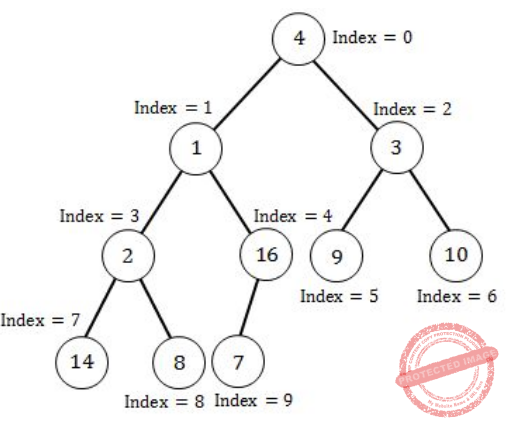Step 2: Since the above heap is not a max heap so to convert a heap into max-heap start applying max- heapify operation from the largest index parent node(node having 1 or more children).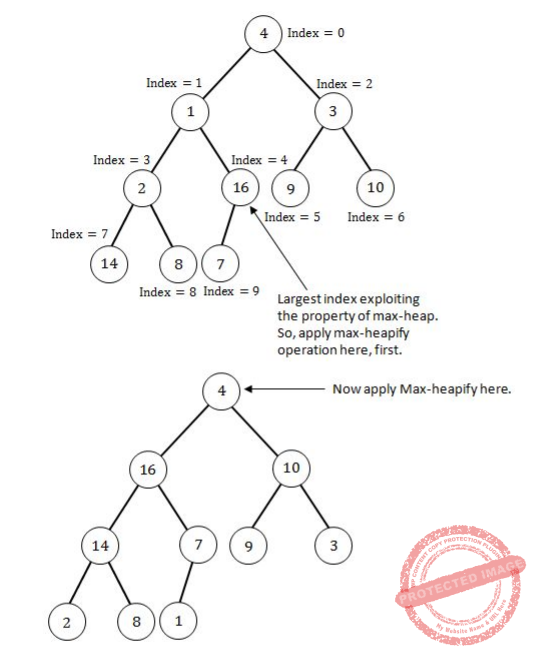The above Heap is the max-heap where each root node have maximum value. Now depth of the Max-heap is 3 and right child of the Root node is 10.
 Question 23
A hash function h defined h(key)=key mod 7, with linear probing, is used to insert the keys 44, 45, 79, 55, 91, 18, 63 into a table indexed from 0 to 6. What will be the location of key 18 ?
 A 3 B 4 C 5 D 6
Data-Structures       Hashing
Question 23 Explanation:
h(key)=key mod 7 is the given hash function and it is mentioned that linear probing is used.
h(44)=44 mod 7 ⇒ 2
h(45)=45 mod 7 ⇒ 3
h(79)=79 mod 7 ⇒ 2 (collision occurred)
h(79)=(79+1) mod 7 ⇒ 3 (collision occurred)
h(79)=(79+2) mod 7 ⇒ 4
h(55)=55 mod 7 ⇒ 6
h(91)=91 mod 7 ⇒ 0
h(18)=18 mod 7 ⇒ 4 (collision occurred)
h(79)=(18+1) mod 7 ⇒ 5
h(63)=63 mod 7 ⇒ 0 (collision occurred)
h(63)=(63+1) mod 7 ⇒ 1
Now the array contain keys 44, 45, 79, 55, 91, 18, 63 at locationsQuestion 24
Which of the following algorithms solves the single-source shortest paths ?
 A Prim’s algorithm B Floyd - Warshall algorithm C Johnson’s algorithm D Dijkstra’s algorithm
Algorithms       Greedy-approach
Question 24 Explanation:
Dijkstra’s algorithm is a Single source shortest path algorithm used to find the shortest path using Greedy approach.
 Question 25
A text is made up of the characters A, B, C, D, E each occurring with the probability 0.08, 0.40, 0.25, 0.15 and 0.12 respectively. The optimal coding technique will have the average length of :
 A 2.4 B 1.87 C 3 D 2.15
Algorithms       Greedy-approach
Question 25 Explanation:Step 1: Select two characters with smallest probabilities and merge them.Step 2: Select two characters from Step 1 with smallest probabilities and merge them.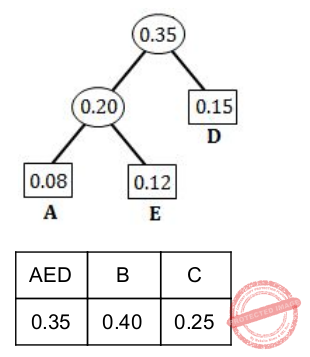Step 3: Select two characters (from Step 2) with smallest probabilities and merge them.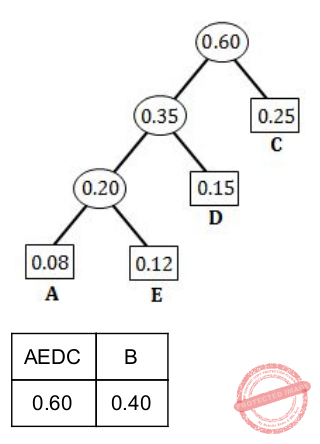Step 4: Merge the remaining two probabilities.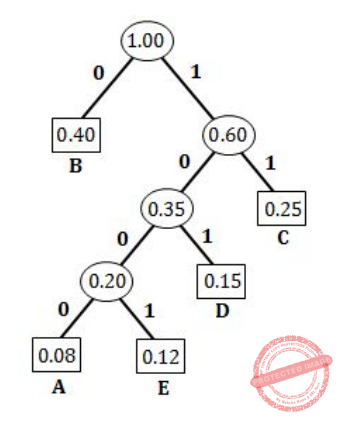A = 1000 (4-bits)
E = 1001 (4-bits)
D = 101 (3-bits)
C = 11 (2-bits)
B = 0 (1-bit)
Average length = ((0.08×4)+(0.12×4)+(0.15×3)+(0.25×2)+(0.40×1)) / (0.08+0.12+0.15+0.25+0.40)
=2.15/1.0
= 2 .15
There are 25 questions to complete.

Register Now